Author: Oscar Cronquist Article last updated on September 05, 2021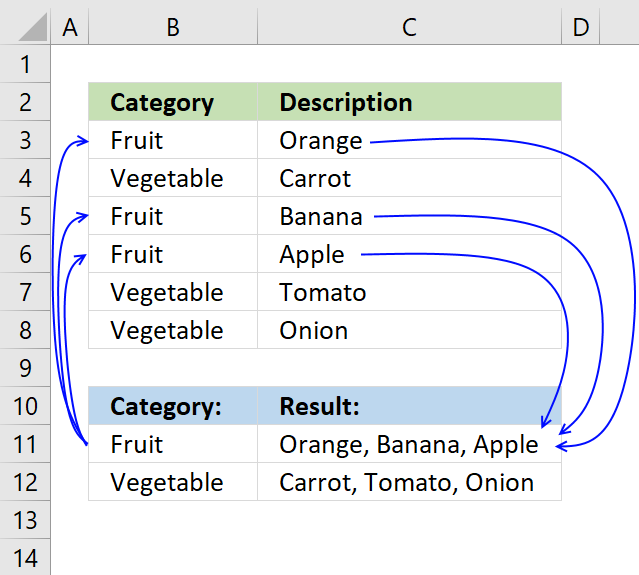This article demonstrates how to find a value in a column and concatenate corresponding values on the same row. The picture above shows an array formula in cell F3 that looks for value "Fruit" in column B and concatenates corresponding values in column C.

The TEXTJOIN function introduced in Excel 2019 allows you to easily concatenate values, it also accepts arrays and nested functions.

However if your Excel version is missing the TEXTJOIN function you can use a User Defined Function, I have all instructions on how to do that in this post.

## 1. Lookup and return multiple values concatenated into one cell [Excel 2019]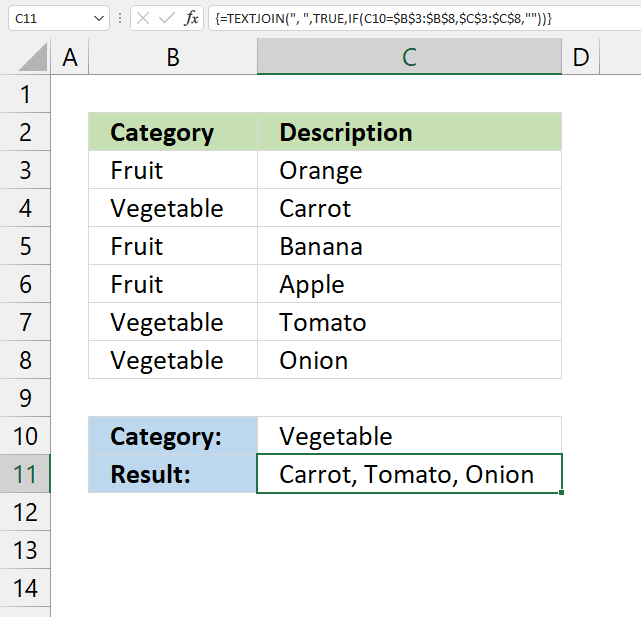The image above demonstrates a formula that returns values concatenated based on a condition. The condition is specified in cell C10, if the condition is met in column B the corresponding value from column C on the same row is extracted and concatenated together.

For example, condition "Vegetable" is found in cells B4, B7, and B8. The corresponding values in cells C4, C7, and C8 are "Carrot", "Tomato", and "Onion". They are concatenated by the TEXTJOIN function that was introduced in Excel 2019.

Array formula in cell C11:

=TEXTJOIN(", ", TRUE, IF(B11=B3:B8, C3:C8, ""))

### 1.1 How to change delimiting character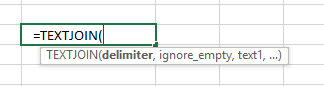The first argument in the TEXTJOIN function lets you specify the delimiting character, I am using ", " in this example.

TEXTJOIN(delimiterignore_emptytext1[text2], ...)

### 1.3 How to enter an array formula

Note, Excel 365 users don't need to enter this formula as an array formula. Dynamic array formulas are entered as regular formulas.

Make sure you enter it as an array formula, follow this:

1. Doublepress with left mouse button on cell C11
2. Paste above formula to cell
3. Press and hold CTRL + SHIFT simultaneously
4. Press enter once
5. Release all keys

If you did this the right way, the formula now has a beginning and ending curly bracket, like this:

{=TEXTJOIN(" ",TRUE,IF(A2='Vehicle applications'!\$C\$2:\$C\$13,'Vehicle applications'!\$A\$2:\$A\$13,""))}

Dont enter these characters yourself, they appear automatically if you follow the instructions above.

Then copy cell C2 and paste to cell range C3:C6.

### 1.4 Explaining formula in cell C11

#### Step 1 - Logical expression

The equal sign is a logical operator that allows you to compare value to value. The result is always a boolean value.

B11=B3:B8

becomes

"Vegetable"={"Fruit"; "Vegetable"; "Fruit"; "Fruit"; "Vegetable"; "Vegetable"}

and returns

{FALSE; TRUE; FALSE; FALSE; TRUE; TRUE}

#### Step 2 - Filter values

The IF function returns one value if the logical expression returns TRUE and another value if the logical expression returns FALSE.

The TEXTJOIN function can ignore empty values if we want to, we can configure the IF function to return empty values if the logical expression returns False.

IF(B11=B3:B8, C3:C8, "")

becomes

IF({FALSE; TRUE; FALSE; FALSE; TRUE; TRUE}, {"Orange"; "Carrot"; "Banana"; "Apple"; "Tomato"; "Onion"; "Apple"; "Carrot"}, "")

and returns

{""; "Carrot"; ""; ""; "Tomato"; "Onion"}

#### Step 3 - Filter values

The TEXTJOIN function allows you to combine text strings from multiple cell ranges and also use delimiting characters if you want.

TEXTJOIN(delimiterignore_emptytext1[text2], ...)

TEXTJOIN(" ", TRUE, IF(B11=B3:B8, C3:C8, ""))

becomes

TEXTJOIN(" ", TRUE, {""; "Carrot"; ""; ""; "Tomato"; "Onion"})

and returns "Carrot, Tomato, Onion" in cell C11.

Here is another example:

Looking for a formula that will take a part number from one column and go and look for all related vehicle applications per that part number and return the vehicle applications to a single cell related back to the part number.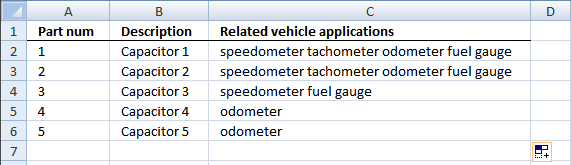Array formula in cell C2:

=TEXTJOIN(" ",TRUE,IF(A2='Vehicle applications'!\$C\$2:\$C\$13,'Vehicle applications'!\$A\$2:\$A\$13,""))

## 2. Lookup and return multiple values concatenated into one cell (UDF)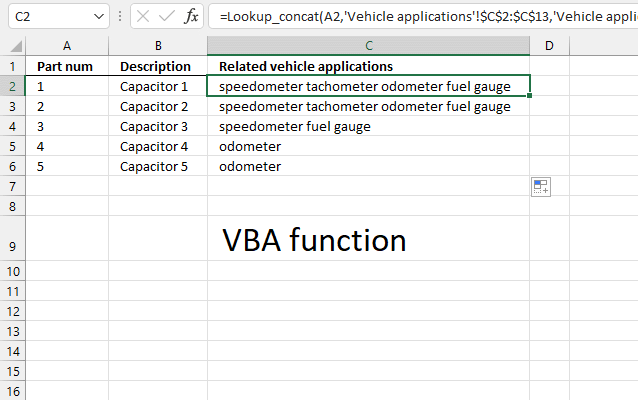UPDATE: The solution below is for Excel versions that have the TEXTJOIN function missing.

User Defined Function in cell C2:

=Lookup_concat(A2, 'Vehicle applications'!\$C\$2:\$C\$13, 'Vehicle applications'!\$A\$2:\$A\$13)

Picture below of worksheet "Vehicle applications".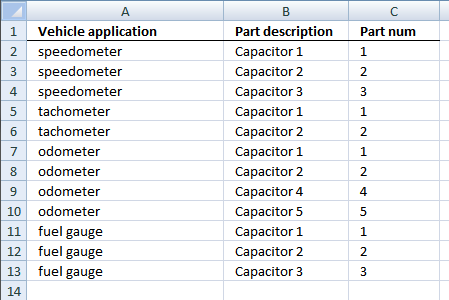Cell A1 (first picture above) contains "1" which is found in cells C2, C5, C7, and C11 (see picture above). The corresponding values on the same row from column A are "speedometer", "tachometer", "odometer, and  "fuel gauge".

### 2.2 User defined function Syntax

Lookup_concat(look_up_value, search_in_column, concatenate_values_in_column)

Looks for a value in a column and returns a value on the same row from a column you specify. If multiple values are found the corresponding values are concatenated into a single cell.

See the picture below.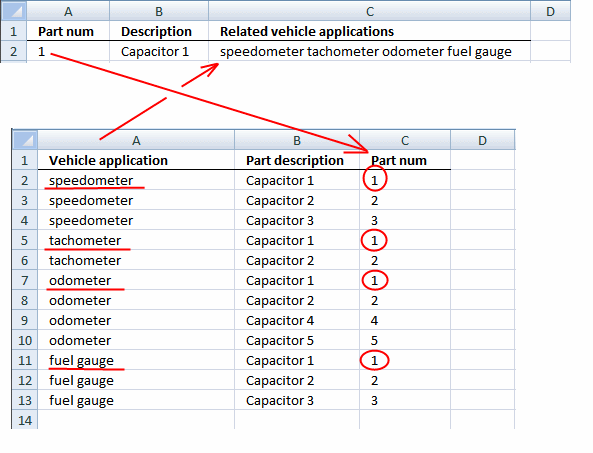### 2.3 VBA code

```'Name user defined function and define parameters
Function Lookup_concat(Search_string As String, _
Search_in_col As Range, Return_val_col As Range)

'Dimension variables and declare data types
Dim i As Long
Dim result As String

'Iterate through each cell in search column
For i = 1 To Search_in_col.Count

'Check if cell is equal to search string
If Search_in_col.Cells(i, 1) = Search_string Then

'Concatenate corresponding value on the same row to the result variable
result = result & " "  Return_val_col.Cells(i, 1).Value
End If

'Continue with next cell
Next

'Return variable to worksheet
Lookup_concat = Trim(result)

End Function
```

### 2.4 Where to put the VBA code?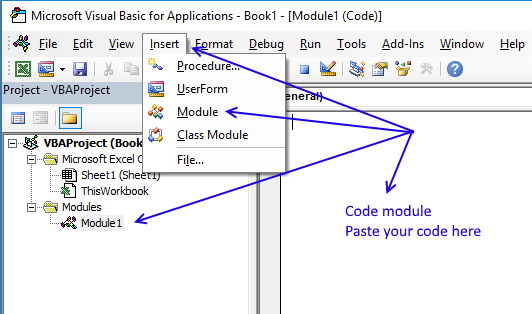1. Press Alt-F11 to open the Visual Basic Editor.
2. Press the left mouse button on "Insert" located on the top menu, see the image above.
3. Press left mouse button on "Module" on the Insert menu to create a new module in your workbook.
4. Copy code above and paste to the code module, see the image above.
6. Save your workbook as a *.xlsm file to keep the code attached to your workbook.

### Get the Excel file## 3. Ignore duplicates - Excel 2019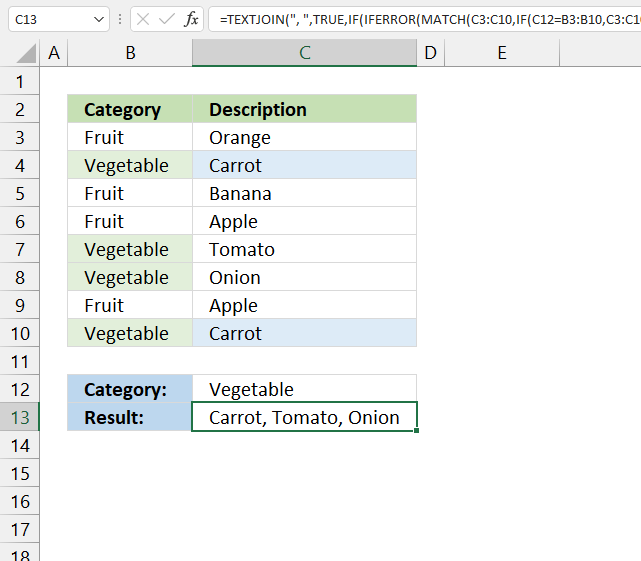This example demonstrates how to ignore duplicate values in the output, it uses the TEXTJOIN function which was introduced in Excel 2019.

The picture below shows you "Carrot" found twice in the table but is only displayed once in cell C13. In other words, no duplicates are allowed in the output.

Array formula in cell C2:

=TEXTJOIN(", ", TRUE, IF(IFERROR(MATCH(C3:C10, IF(C12=B3:B10, C3:C10, ""), 0), "")=MATCH(ROW(B3:B10), ROW(B3:B10)), C3:C10, ""))

Watch a video where I explain the formula

How to enter an array formula

### 3.1 Explaining array formula in cell C13

#### Step 1 - Logical expression

The equal sign is a logical operator that lets you to compare value to value, the output is a boolean value.

In this case, the value in cell C12 is compared to all values in cell range B3:B10. The logical expression returns an array of boolean values.

C12=B3:B10

becomes

"Vegetable"={"Fruit"; "Vegetable"; "Fruit"; "Fruit"; "Vegetable"; "Vegetable"; "Fruit"; "Vegetable"}

and returns

{FALSE; TRUE; FALSE; FALSE; TRUE; TRUE; FALSE; TRUE}.

#### Step 2 - Filter values

The IF function returns one value if the logical expression returns TRUE and another value if the logical expression returns FALSE.

IF(logical_test, [value_if_true], [value_if_false])

IF(C12=B3:B10, C3:C10, "")

becomes

IF({FALSE; TRUE; FALSE; FALSE; TRUE; TRUE; FALSE; TRUE}, {"Orange"; "Carrot"; "Banana"; "Apple"; "Tomato"; "Onion"; "Apple"; "Carrot"}, "")

and returns

{""; "Carrot"; ""; ""; "Tomato"; "Onion"; ""; "Carrot"}.

#### Step 3 - Match values

The MATCH function returns the relative position of an item in an array or cell reference that matches a specified value in a specific order.

MATCH(lookup_value, lookup_array, [match_type])

MATCH(C3:C10, IF(C12=B3:B10, C3:C10, ""), 0)

becomes

MATCH({"Orange"; "Carrot"; "Banana"; "Apple"; "Tomato"; "Onion"; "Apple"; "Carrot"}, {""; "Carrot"; ""; ""; "Tomato"; "Onion"; ""; "Carrot"}, 0)

and returns {#N/A; 2; #N/A; #N/A; 5; 6; #N/A; 2}.

The MATCH function returns an error #N/A if the lookup_value is not found in the lookup_array, we will take care of this in the next step.

#### Step 4 - Remove errors

The IFERROR function lets you catch most errors in Excel formulas. We will replace the error value with an empty value.

IFERROR(valuevalue_if_error)

IFERROR(MATCH(C3:C10, IF(C12=B3:B10, C3:C10, ""), 0), "")

becomes

IFERROR({#N/A; 2; #N/A; #N/A; 5; 6; #N/A; 2}, "")

and returns {""; 2; ""; ""; 5; 6; ""; 2}.

#### Step 5 - Create sequence

The ROW function calculates the row number based on a cell reference. We use a cell reference that points to a cell range instead of a single cell. This returns an array of numbers.

ROW(reference)

ROW(B3:B10)

returns {3; 4; 5; 6; 7; 8; 9; 10}.

#### Step 6 - Create a number sequence from 1 to n

MATCH(ROW(B3:B10), ROW(B3:B10))

becomes

MATCH({3; 4; 5; 6; 7; 8; 9; 10}, {3; 4; 5; 6; 7; 8; 9; 10})

and returns {1; 2; 3; 4; 5; 6; 7; 8}.

#### Step 7 - Compare arrays

This step compares the arrays which are of the same size, both contain the same number of values.

This allows us to identify duplicate values, a number that doesn't match the sequence is a duplicate or a blank value.

MATCH(C3:C10, IF(C12=B3:B10, C3:C10, ""), 0), "")=MATCH(ROW(B3:B10), ROW(B3:B10))

becomes

{""; 2; ""; ""; 5; 6; ""; 2}={1; 2; 3; 4; 5; 6; 7; 8}

and returns

{FALSE; TRUE; FALSE; FALSE; TRUE; TRUE; FALSE; FALSE}.

#### Step 8 - Filter unique distinct values

This step creates an array that contains only unique distinct values, in other words, no duplicates.

IF(IFERROR(MATCH(C3:C10, IF(C12=B3:B10, C3:C10, ""), 0), "")=MATCH(ROW(B3:B10), ROW(B3:B10)), C3:C10, "")

becomes

IF({FALSE; TRUE; FALSE; FALSE; TRUE; TRUE; FALSE; FALSE}, C3:C10, "")

and returns

{""; "Carrot"; ""; ""; "Tomato"; "Onion"; ""; ""}.

#### Step 9 - Concatenate unique distinct values

The TEXTJOIN function allows you to combine text strings from multiple cell ranges and also use delimiting characters if you want.

TEXTJOIN(delimiterignore_emptytext1[text2], ...)

TEXTJOIN(", ", TRUE, IF(IFERROR(MATCH(C3:C10, IF(C12=B3:B10, C3:C10, ""), 0), "")=MATCH(ROW(B3:B10), ROW(B3:B10)), C3:C10, ""))

becomes

TEXTJOIN(", ", TRUE, {""; "Carrot"; ""; ""; "Tomato"; "Onion"; ""; ""})

and returns "Carrot, Tomato, Onion" in cell C13.

## 4. Ignore duplicates - Excel 365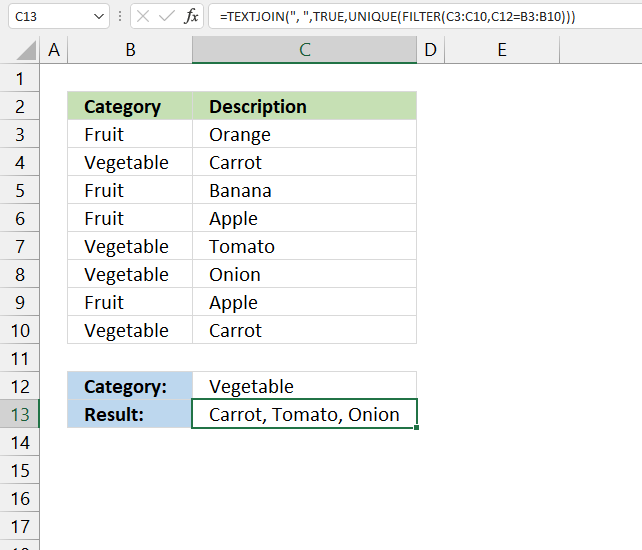This formula is a dynamic array formula and works only in Excel 365, it is entered as a regular formula. Both the FILTER and UNIQUE functions are new functions in Excel 365.

These new functions make the formula considerably smaller and easier to understand.

Formula in cell C13:

=TEXTJOIN(", ", TRUE, UNIQUE(FILTER(C3:C10, C12=B3:B10)))

### 4.1 Explaining formula in cell C13

#### Step 1 - Logical test

The equal sign is a logical operator that lets you compare value to value. The result is always a boolean value.

In this case, the value in cell C12 is compared to all values in cell range B3:B10. The logical expression returns an array of boolean values.

C12=B3:B10

becomes

"Vegetable"={"Fruit"; "Vegetable"; "Fruit"; "Fruit"; "Vegetable"; "Vegetable"; "Fruit"; "Vegetable"}

and returns

{FALSE; TRUE; FALSE; FALSE; TRUE; TRUE; FALSE; TRUE}.

#### Step 2 - Filter values

The FILTER function lets you extract values/rows based on a condition or criteria.

FILTER(arrayinclude, [if_empty])

FILTER(C3:C10,C12=B3:B10)

becomes

FILTER({"Orange"; "Carrot"; "Banana"; "Apple"; "Tomato"; "Onion"; "Apple"; "Carrot"},{FALSE; TRUE; FALSE; FALSE; TRUE; TRUE; FALSE; TRUE})

and returns

{"Carrot"; "Tomato"; "Onion"; "Carrot"}.

#### Step 3 - Create a unique distinct list

The UNIQUE function lets you extract both unique and unique distinct values and also comparing columns to columns or rows to rows.

UNIQUE(FILTER(C3:C10,C12=B3:B10))

becomes

UNIQUE({"Carrot"; "Tomato"; "Onion"; "Carrot"})

and returns

{"Carrot";"Tomato";"Onion"}.

#### Step 4 - Concatenate values

The TEXTJOIN function allows you to combine text strings from multiple cell ranges and also use delimiting characters if you want.

TEXTJOIN(delimiterignore_emptytext1[text2], ...)

TEXTJOIN(", ",TRUE,UNIQUE(FILTER(C3:C10,C12=B3:B10)))

becomes

TEXTJOIN(", ",TRUE,{"Carrot";"Tomato";"Onion"})

and returns "Carrot, Tomato, Onion".

## 5. Ignore duplicates (UDF)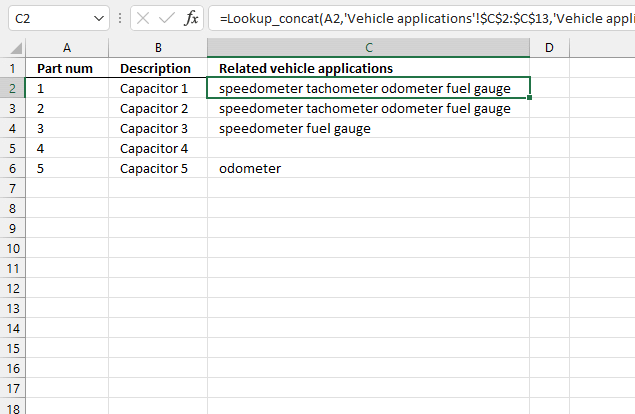The image above shows a User Defined Function in cell C2 that lookup and returns multiple concatenated unique distinct values.

Formula in cell C2:

=Lookup_concat(A2, 'Vehicle applications'!\$C\$2:\$C\$13, 'Vehicle applications'!\$A\$2:\$A\$13)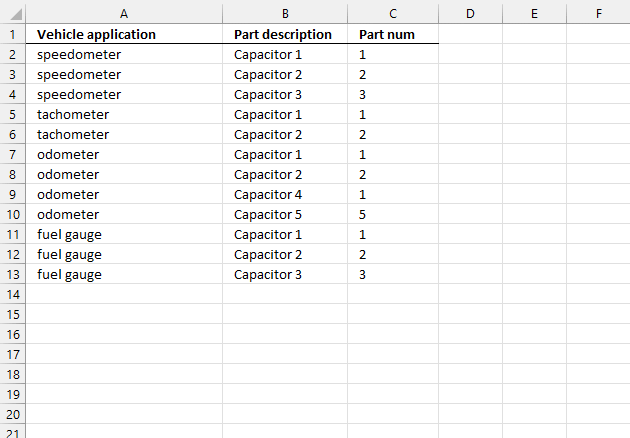The image above shows worksheet "Vehicle applications". The part number in cell A2 matches cells C2, C5, C7, C9, and C11. Cell C7 and C9 are duplicates, however, the UDF returns only one instance of each value.

### 5.1 Video

Watch a video where I explain how to use the UDF:

### 5.2 VBA code

You need to add two User Defined Functions to your workbook. They let you return unique distinct values concatenated into one cell, one UDF creates a unique distinct list and the other UDF concatenates the values.

```'Name User Defined Function and define parameters
Function Lookup_concat(Search_string As String, _
Search_in_col As Range, Return_val_col As Range)

'Dimension variables and declare data types
Dim i As Long
Dim temp() As Variant
Dim result As String

'Create an array variable
ReDim temp(0)

'Iterate through all cells in the search range
For i = 1 To Search_in_col.Count

'Check if cell value equals search string
If Search_in_col.Cells(i, 1) = Search_string Then

'Add value to array if true
temp(UBound(temp)) = Return_val_col.Cells(i, 1).Value

'Add another container to array variable
ReDim Preserve temp(UBound(temp) + 1)
End If
Next

'Check if first value in array variable is not equal to nothing
If temp(0) <> "" Then

'Remove container from array variable
ReDim Preserve temp(UBound(temp) - 1)

'Start User defined function named Unique with parameter temp which contains values
Unique temp

'Iterate through array variable temp
For i = LBound(temp) To UBound(temp)

'Add each value in array variable temp to variable result and add a delimiting character
result = result & " " & temp(i)

'Continue with next value
Next i

'Remove leading and trailing blanks and then return string to worksheet
Lookup_concat = Trim(result)

'Continue here if first value in temp array is nothing
Else

'Return blank to worksheet, this avoids an error being returned when no values match the condition
Lookup_concat = ""
End If

End Function
```

The following UDF returns a unique distinct list

```'Name user defined function and define parameter
Function Unique(tempArray As Variant)

'Dimension variables and declare data types
Dim coll As New Collection
Dim Value As Variant

'Enable error handling
On Error Resume Next

'Iterate through each value in tempArray
For Each Value In tempArray

'Check if value has more characters than 0 (zero), if so add string to collection coll
If Len(Value) <> 0 Then coll.Add Value, CStr(Value)

'Continue with next value
Next Value

'Disable error handling
On Error GoTo 0

'Clear array variable tempArray
ReDim tempArray(0)

'Iterate through each value stored in collection variable coll
For Each Value In coll

'Save value to array variable tempArray
tempArray(UBound(tempArray)) = Value

'Add another container to array variable tempArray
ReDim Preserve tempArray(UBound(tempArray) + 1)

'Continue with next value
Next Value

End Function

```

### Get the Excel filelookup-and-return-multiple-concatenated-unique-distinct-values.xlsm

## 6. Change delimiting character (UDF)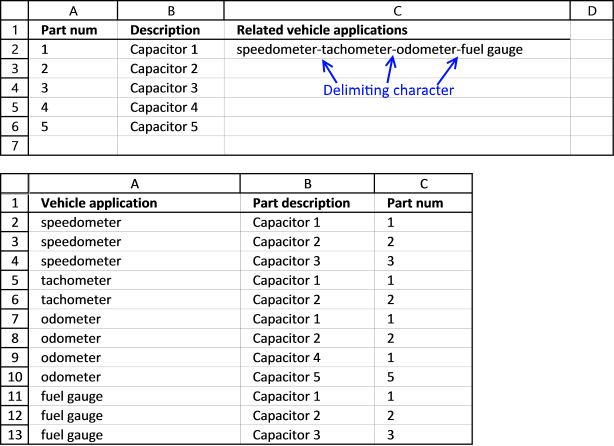This UDF lets you specify a delimiting character in the last argument.

Formula in cell C2:

=Lookup_concat(A2,'Vehicle applications'!\$C\$2:\$C\$13,'Vehicle applications'!\$A\$2:\$A\$13,"-")

The last argument in the User Defined Function lets you specify a delimiting string, it can be one character it can be many.

### 6.2 VBA Code

```'Name user defined function and define parameters
Function Lookup_concat(Search_string As String, _
Search_in_col As Range, Return_val_col As Range, str As String)

'Dimension variables and declare data types
Dim i As Long
Dim result As String

'Iterate through each cell in search range
For i = 1 To Search_in_col.Count

'Check if cell value matches search string
If Search_in_col.Cells(i, 1) = Search_string Then

'Add value to result variable and delimting character specified in parameter str
result = result & str & Return_val_col.Cells(i, 1).Value
End If

'Continue with next cell
Next

'Check if the number of characters in result variable is larger than 0 (zero)
If Len(result) > 0 Then

'Remove last delmiting string
result = Right(result, Len(result) - Len(str))

'Return values to worksheet
Lookup_concat = Trim(result)

'Continue here if the number of characters in result variable is not larger than 0 (zero)
Else

'Return nothing to worksheet
Lookup_concat = ""
End If
End Function

```

### Get the Excel filelookup-and-return-multiple-concatenated-values-delimiting-character.xlsm

## 7. Match if cell contains string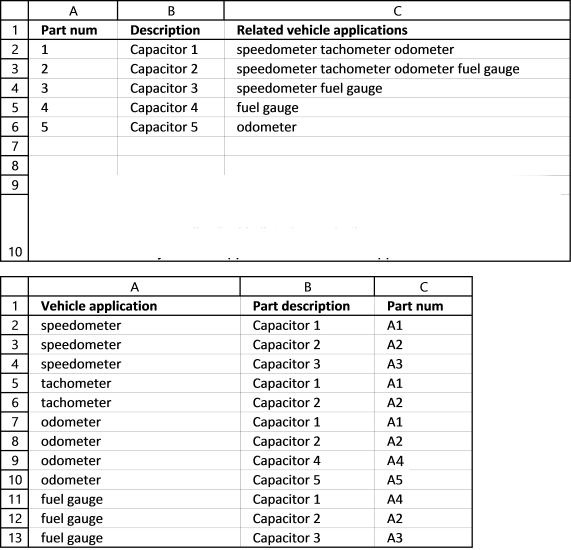This example demonstrates how to concatenate values if corresponding values on the same row contain a specific string.

The first image above shows the array formula in cell C2:

=TEXTJOIN(",",TRUE,IFERROR(IF(SEARCH(A2,'Vehicle applications'!\$C\$2:\$C\$13),'Vehicle applications'!\$A\$2:\$A\$13,""),""))

The way this works is that the formula in cell C2 checks if the value in cell A2 matches a string in cell range C2:C13. In other words, the whole cell is not required to match but a sub-string must.

Search string "1" is found in cell C2,C5 and C7 in the second image shown above, the corresponding values are "speedometer", "tachometer", and "odometer". Those values are returned to cell C2 shown in the first image above.

Watch a video where I explain the formula

How to enter an array formula

### 7.1 Explaining array formula in cell C2

#### Step 1 - Search for string in cell range

The SEARCH function returns a number representing the position of character at which a specific text string is found reading left to right. It is not a case-sensitive search.

SEARCH(A2,'Vehicle applications'!\$C\$2:\$C\$13)

becomes

SEARCH(1,{"A1"; "A2"; "A3"; "A1"; "A2"; "A1"; "A2"; "A4"; "A5"; "A4"; "A2"; "A3"})

and returns

{2; #VALUE!; #VALUE!; 2; #VALUE!; 2; #VALUE!; #VALUE!; #VALUE!; #VALUE!; #VALUE!; #VALUE!}

#### Step 2 - Return adjacent value

The IF function returns one value if the logical test is TRUE and another value if the logical test is FALSE.

IF(logical_test, [value_if_true], [value_if_false])

IF(SEARCH(A2,'Vehicle applications'!\$C\$2:\$C\$13),'Vehicle applications'!\$A\$2:\$A\$13,"")

becomes

IF({2; #VALUE!; #VALUE!; 2; #VALUE!; 2; #VALUE!; #VALUE!; #VALUE!; #VALUE!; #VALUE!; #VALUE!},'Vehicle applications'!\$A\$2:\$A\$13,"")

becomes

IF({2;  #VALUE!;  #VALUE!;  2;  #VALUE!;  2;  #VALUE!;  #VALUE!;  #VALUE!;  #VALUE!;  #VALUE!;  #VALUE!},{"speedometer"; "speedometer"; "speedometer"; "tachometer"; "tachometer"; "odometer"; "odometer"; "odometer"; "odometer"; "fuel gauge"; "fuel gauge"; "fuel gauge"},"")

and returns

{"speedometer"; #VALUE!; #VALUE!; "tachometer"; #VALUE!; "odometer"; #VALUE!; #VALUE!; #VALUE!; #VALUE!; #VALUE!; #VALUE!}

#### Step 3 - Remove errors

The IFERROR function lets you catch most errors in Excel formulas.

IFERROR(valuevalue_if_error)

IFERROR(IF(SEARCH(A2,'Vehicle applications'!\$C\$2:\$C\$13),'Vehicle applications'!\$A\$2:\$A\$13,""),"")

becomes

IFERROR({"speedometer"; #VALUE!; #VALUE!; "tachometer"; #VALUE!; "odometer"; #VALUE!; #VALUE!; #VALUE!; #VALUE!; #VALUE!; #VALUE!},"")

and returns

{"speedometer"; ""; ""; "tachometer"; ""; "odometer"; ""; ""; ""; ""; ""; ""}.

#### Step 4 - Concatenate values

The TEXTJOIN function allows you to combine text strings from multiple cell ranges and also use delimiting characters if you want.

TEXTJOIN(delimiterignore_emptytext1[text2], ...)

TEXTJOIN(",",TRUE,IFERROR(IF(SEARCH(A2,'Vehicle applications'!\$C\$2:\$C\$13),'Vehicle applications'!\$A\$2:\$A\$13,""),""))

becomes

TEXTJOIN(",",TRUE,{"speedometer";"";"";"tachometer";"";"odometer";"";"";"";"";"";""})

and returns "speedometer, tachometer, odometer" in cell C2.

## 8. Match if cell contains string - User Defined Function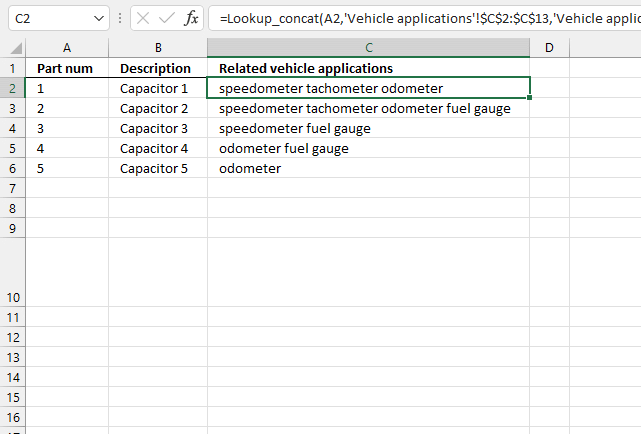This example demonstrates a User Defined Function that matches cells that contain the search string and returns values from the same row concatenated, no duplicates are returned.

Formula in cell C2:

=Lookup_concat(A2, 'Vehicle applications'!\$C\$2:\$C\$13, 'Vehicle applications'!\$A\$2:\$A\$13)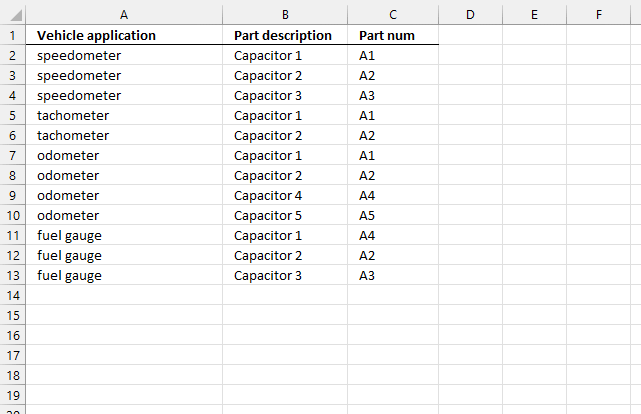Cell A2 (first picture above) contains "1" which is found in cells C2, C5, and C7 (image above). The corresponding cells on the same row are concatenated and returned to cell C2 in the first picture above.

### 8.1 Video

For those of you that have an earlier Excel version. Watch this video where I explain how to use it:

### 8.2 VBA Code

```'Name user defined function and define parameters
Function Lookup_concat(Search_string As String, _
Search_in_col As Range, Return_val_col As Range)

'Dimension variables and declare data types
Dim i As Long
Dim temp() As Variant
Dim result As String

'Create array variable
ReDim temp(0)

'Iterate through search range
For i = 1 To Search_in_col.Count

'Check if cell contains search string
If InStr(UCase(Search_in_col.Cells(i, 1)), UCase(Search_string)) Then

'Save cell value to array variable temp if line above is true
temp(UBound(temp)) = Return_val_col.Cells(i, 1).Value

'Add another container to array variable temp
ReDim Preserve temp(UBound(temp) + 1)
End If

'Continue with next cell
Next

'Check if first value in array variable temp is not equal to nothing
If temp(0) <> "" Then

'Remove last container in array variable temp
ReDim Preserve temp(UBound(temp) - 1)

'Iterate through values in array variable temp
For i = LBound(temp) To UBound(temp)

'Concatenate value to variable result and a delimiting character " "
result = result & " " & temp(i)

'Continue with next value
Next i

'Remove leading and trailing spaces and then return result variable to worksheet
Lookup_concat = Trim(result)

'Continue here if first value in array variable temp is equal to nothing
Else

'Return nothing to worksheet
Lookup_concat = ""
End If

End Function

```

### Get the Excel fileexcel-vba-return-multiple-unique-values-into-one-cell-wildcard-searchv2.xlsm

## 9. Searching for the first characters in a text string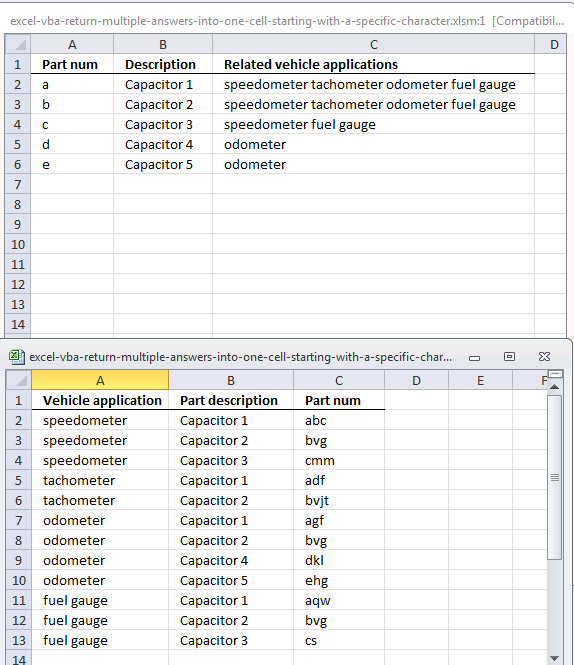The formula and UDF demonstrated in the picture above look for a string that begins with the search string. If the search string is "a" it will match "ab", "ac" but not "ba". "ab" and "ac" begin with an "a".

If the search string is "ab" it will match "abc", "abz" but not "bca".

Array formula in cell C2:

=TEXTJOIN(",", TRUE, IF(A2=LEFT('Vehicle applications'!\$C\$2:\$C\$13, LEN(A2)), 'Vehicle applications'!\$A\$2:\$A\$13, ""))

Watch a video where I explain the formula

How to enter an array formula

### 9.1 Explaining array formula in cell C2

#### Step 1 - Crop strings so they match search string length

The LEFT function extracts a specific number of characters always starting from the left.

LEFT(text, [num_chars])

LEFT('Vehicle applications'!\$C\$2:\$C\$13, LEN(A2)

becomes

LEFT({"abc"; "bvg"; "cmm"; "adf"; "bvjt"; "agf"; "bvg"; "dkl"; "ehg"; "aqw"; "bvg"; "cs"}, LEN("a")

and returns

{"a"; "b"; "c"; "a"; "b"; "a"; "b"; "d"; "e"; "a"; "b"; "c"}

#### Step 2 - Check if "a" is equal to values in array

The IF function returns one value if the logical expression returns TRUE and another value if the logical expression returns FALSE.

IF(logical_test, [value_if_true], [value_if_false])

IF(A2=LEFT('Vehicle applications'!\$C\$2:\$C\$13, LEN(A2)), 'Vehicle applications'!\$A\$2:\$A\$13, "")

becomes

IF("a"={"a"; "b"; "c"; "a"; "b"; "a"; "b"; "d"; "e"; "a"; "b"; "c"},'Vehicle applications'!\$A\$2:\$A\$13,"")

and returns

{"speedometer"; ""; ""; "tachometer"; ""; "odometer"; ""; ""; ""; "fuel gauge"; ""; ""}

#### Step 3 - Concatenate values

The TEXTJOIN function allows you to combine text strings from multiple cell ranges and also use delimiting characters if you want.

TEXTJOIN(delimiterignore_emptytext1[text2], ...)

TEXTJOIN(",", TRUE, IF(A2=LEFT('Vehicle applications'!\$C\$2:\$C\$13, LEN(A2)), 'Vehicle applications'!\$A\$2:\$A\$13, ""))

becomes

TEXTJOIN(", ", TRUE, {"speedometer";"";"";"tachometer";"";"odometer";"";"";"";"fuel gauge";"";""})

and returns

"speedometer, tachometer, odometer, fuel gauge" in cell C2.

## 10. Searching for the first characters in a text string [UDF]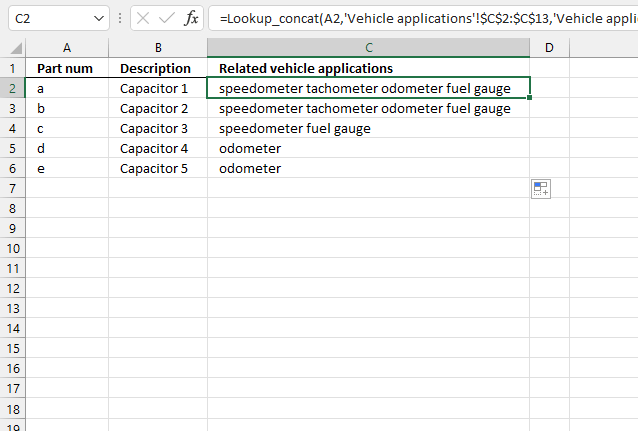User Defined Function in cell C2:

=Lookup_concat(A2, 'Vehicle applications'!\$C\$2:\$C\$13, 'Vehicle applications'!\$A\$2:\$A\$13)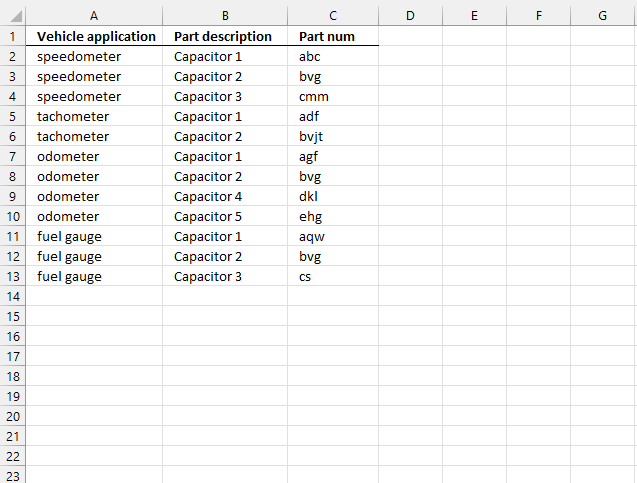The formula in cell C2, demonstrated in the first image above, concatenates values from column A if the corresponding value in column C  (second image above) begins with the value specified in cell A2 (first image).

Cell C2, C5, C7, and C11 have values that begin with an "a", the formula in cell C2 returns these values "speedometer tachometer odometer fuel gauge" extracted from column A on the same rows as cells C2, C5, C7, and C11.

### 10.1 VBA code

```'Name User defined Function and define parameters
Function Lookup_concat(Search_string As String, _
Search_in_col As Range, Return_val_col As Range)

'Dimension variables and declare data types
Dim i As Long
Dim result As String

'Iterate through all cells in search range
For i = 1 To Search_in_col.Count

'Check if cell begins with search string
If Left(Search_in_col.Cells(i, 1), Len(Search_string)) = Search_string Then

'Concatenate return value with variable result if search range value begins with search string
result = result & " " & Return_val_col.Cells(i, 1).Value
End If
Next

'Return variable result without leading and trailing space characters to worksheet
Lookup_concat = Trim(result)

End Function

```

### Get the Excel file## 11. Lookup and return multiple dates concatenated into one cell (formula)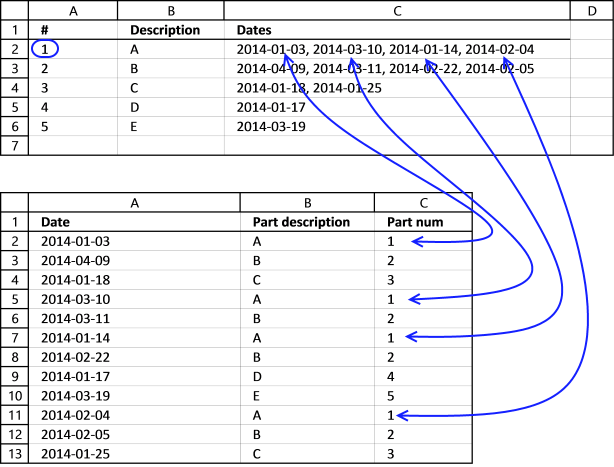This example demonstrates a formula that concatenates dates based on a condition. The condition is specified in cell A2 shown in the first worksheet above.

The picture shows dates formatted in this order: YYYY-MM-DD, don't worry you can change this.

Array formula in cell C2:

=TEXTJOIN(", ",TRUE,IF(A2='Vehicle applications'!\$C\$2:\$C\$13,TEXT('Vehicle applications'!\$A\$2:\$A\$13,"YYYY-MM-DD"),""))

Excel sees dates as numbers so they can be used in formulas and calculations, Jan 1, 1900 is 1, and June 7, 2017 is 42893. To concatenate dates and not numbers we need to use the TEXT function to convert numbers to dates.

Change date formatting to "MM/DD/YYYY" if you live in the United States.

Watch a video where I explain the formula

### 11.1 Explaining formula in cell C2

#### Step 1 - Format Excel date numbers to dates

The TEXT function converts a value to text in a specific number format, we will use this to convert Excel dates to dates.

TEXT(value, format_text)

TEXT('Vehicle applications'!\$A\$2:\$A\$13,"YYYY-MM-DD")

becomes

TEXT({41642; 41738; 41657; 41708; 41709; 41653; 41692; 41656; 41717; 41674; 41675; 41664},"YYYY-MM-DD")

and returns

{"2014-01-03"; "2014-04-09"; "2014-01-18"; "2014-03-10"; "2014-03-11"; "2014-01-14"; "2014-02-22"; "2014-01-17"; "2014-03-19"; "2014-02-04"; "2014-02-05"; "2014-01-25"}

#### Step 2 - Check if "a" is equal to values in array

The IF function returns one value if the logical test is TRUE and another value if the logical test is FALSE.

IF(logical_test, [value_if_true], [value_if_false])

IF(A2='Vehicle applications'!\$C\$2:\$C\$13,TEXT('Vehicle applications'!\$A\$2:\$A\$13,"YYYY-MM-DD"),"")

becomes

IF(A2='Vehicle applications'!\$C\$2:\$C\$13,{"2014-01-03"; "2014-04-09"; "2014-01-18"; "2014-03-10"; "2014-03-11"; "2014-01-14"; "2014-02-22"; "2014-01-17"; "2014-03-19"; "2014-02-04"; "2014-02-05"; "2014-01-25"},"")

becomes

=IF({TRUE;FALSE;FALSE;TRUE;FALSE;TRUE;FALSE;FALSE;FALSE;TRUE;FALSE;FALSE},{"2014-01-03"; "2014-04-09"; "2014-01-18"; "2014-03-10"; "2014-03-11"; "2014-01-14"; "2014-02-22"; "2014-01-17"; "2014-03-19"; "2014-02-04"; "2014-02-05"; "2014-01-25"},"")

and returns

{"2014-01-03";"";"";"2014-03-10";"";"2014-01-14";"";"";"";"2014-02-04";"";""}

#### Step 3 - Concatenate values

The TEXTJOIN function allows you to combine text strings from multiple cell ranges and also use delimiting characters if you want.

TEXTJOIN(delimiterignore_emptytext1[text2], ...)

TEXTJOIN(", ",TRUE,IF(A2='Vehicle applications'!\$C\$2:\$C\$13,TEXT('Vehicle applications'!\$A\$2:\$A\$13,"YYYY-MM-DD"),""))

becomes

TEXTJOIN(", ",TRUE,{"2014-01-03";"";"";"2014-03-10";"";"2014-01-14";"";"";"";"2014-02-04";"";""})

and returns

"2014-01-03, 2014-03-10, 2014-01-14, 2014-02-04" in cell C2.

## 12. Lookup and return multiple dates concatenated into one cell [UDF]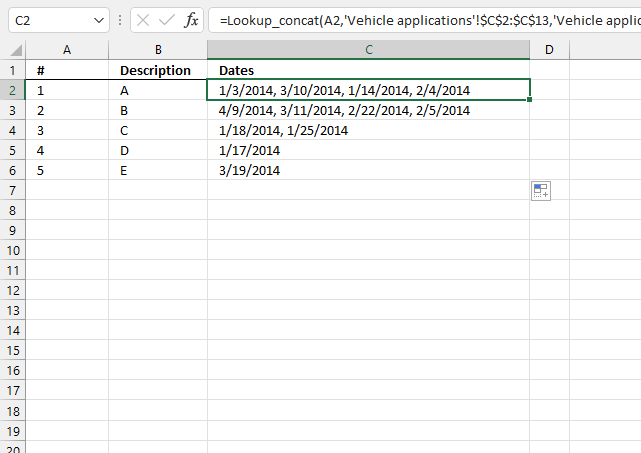This example demonstrates a User Defined Function that extracts and concatenates dates based on a condition applied to values in another column.

User Defined Function in cell C2:

=Lookup_concat(A2, 'Vehicle applications'!\$C\$2:\$C\$13, 'Vehicle applications'!\$A\$2:\$A\$13)

The image below shows worksheet Vehicle applications.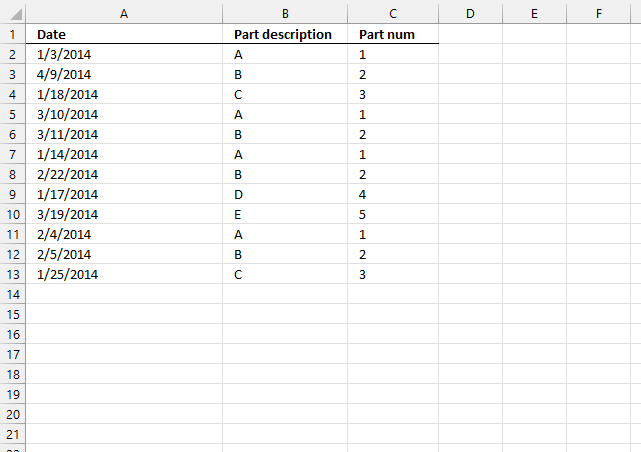The UDF concatenates dates from column A if values in column C on the same row match the condition specified in cell A2 (first image above).

### 12.1 VBA code

```'Name User defined Function and define parameters
Function Lookup_concat(Search_string As String, _
Search_in_col As Range, Return_val_col As Range)

'Dimension variables and declare data types
Dim i As Long
Dim result As String

'Iterate through each cell in search range
For i = 1 To Search_in_col.Count

'Check if cell value matches search string
If Search_in_col.Cells(i, 1) = Search_string Then

'Add value to result variable and delimting character specified in parameter str
result = result & ", " & Return_val_col.Cells(i, 1).Value
End If
Next

'Remove delimiting characters and return string to worksheet
Lookup_concat = Right(result, Len(result) - 2)

End Function

```

### Get the Excel fileexcel-vba-return-multiple-dates-into-one-cell.xlsm

## 13. Lookup within a date range and return multiple values concatenated into one cell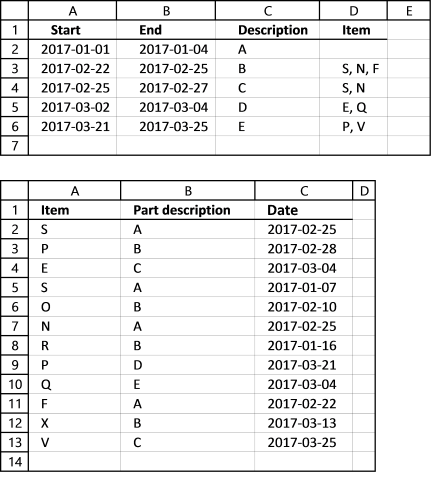The following formula use a start and end date to filter dates in col C (table2) and return corresponding items on the same row in col A to cell D3.

Array formula in cell D3 (first worksheet above):

=TEXTJOIN(", ", TRUE, IF((A3<='Vehicle applications'!\$C\$2:\$C\$13) *(B3>='Vehicle applications'!\$C\$2:\$C\$13), 'Vehicle applications'!\$A\$2:\$A\$13, ""))

Watch a video where I explain the formula

How to enter an array formula

### 13.1 Explaining formula in cell D3

#### Step 1 - Check which dates are larger than or equal to start date

The less than character and the equal sign are logical operators and check if dates are inside the date range. The output is an array of boolean values TRUE or FALSE.

A3<='Vehicle applications'!\$C\$2:\$C\$13

becomes

42788<={42791; 42794; 42798; 42742; 42776; 42791; 42751; 42815; 42798; 42788; 42807; 42819}

and returns

{TRUE; TRUE; TRUE; FALSE; FALSE; TRUE; FALSE; TRUE; TRUE; TRUE; TRUE; TRUE}.

#### Step 2 - Check which dates are smaller than or equal to start date

B3>='Vehicle applications'!\$C\$2:\$C\$13

becomes

42791>={42791; 42794; 42798; 42742; 42776; 42791; 42751; 42815; 42798; 42788; 42807; 42819}

and returns

{TRUE; FALSE; FALSE; TRUE; TRUE; TRUE; TRUE; FALSE; FALSE; TRUE; FALSE; FALSE}.

#### Step 3 - Multiply arrays - AND logic

Both conditions must be true for a date to be in a date range, this means we must apply AND logic meaning:

TRUE * TRUE = TRUE
TRUE * FALSE = FALSE
FALSE * TRUE = FALSE
FALSE * FALSE = FALSE

This requires us to multiply the arrays. Excel converts boolean values to their numerical equivalents when we multiply boolean values.

TRUE = 1 and FALSE = 0 (zero)

(A3<='Vehicle applications'!\$C\$2:\$C\$13) *(B3>='Vehicle applications'!\$C\$2:\$C\$13)

becomes

(42788<={42791; 42794; 42798; 42742; 42776; 42791; 42751; 42815; 42798; 42788; 42807; 42819}) *(42791>={42791; 42794; 42798; 42742; 42776; 42791; 42751; 42815; 42798; 42788; 42807; 42819})

and returns

{1; 0; 0; 0; 0; 1; 0; 0; 0; 1; 0; 0}

#### Step 4 - Return value on same row as matching date

The IF function creates an array containing values from cell range A2:A13 worksheet "Vehicle applications" if the corresponding value in the array is TRUE or 1. If FALSE nothing "" is returned.

IF((A3<='Vehicle applications'!\$C\$2:\$C\$13) *(B3>='Vehicle applications'!\$C\$2:\$C\$13), 'Vehicle applications'!\$A\$2:\$A\$13, "")

becomes

IF({1; 0; 0; 0; 0; 1; 0; 0; 0; 1; 0; 0}, {"S"; "P"; "E"; "S"; "O"; "N"; "R"; "P"; "Q"; "F"; "X"; "V"}, "")

and returns

{"S"; ""; ""; ""; ""; "N"; ""; ""; ""; "F"; ""; ""}

#### Step 5 - Concatenate values

The TEXTJOIN function allows you to combine text strings from multiple cell ranges and also use delimiting characters if you want.

TEXTJOIN(delimiterignore_emptytext1[text2], ...)

TEXTJOIN(", ", TRUE, IF((A3<='Vehicle applications'!\$C\$2:\$C\$13) *(B3>='Vehicle applications'!\$C\$2:\$C\$13), 'Vehicle applications'!\$A\$2:\$A\$13, ""))

becomes

TEXTJOIN(", ", TRUE, {"S"; ""; ""; ""; ""; "N"; ""; ""; ""; "F"; ""; ""})

and returns

"S, N, F" in cell D3.

## 14. Lookup within a date range and return multiple values concatenated into one cell [UDF]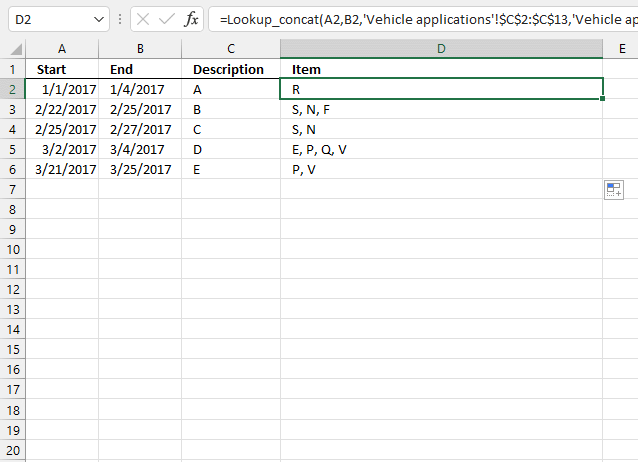The UDF in cell D2 extracts and concatenates dates based on a date range specified in cells A2 and B2.

Formula in cell D2:

=Lookup_concat(A2,B2,'Vehicle applications'!\$C\$2:\$C\$13,'Vehicle applications'!\$A\$2:\$A\$13)

The following image show data on the second worksheet.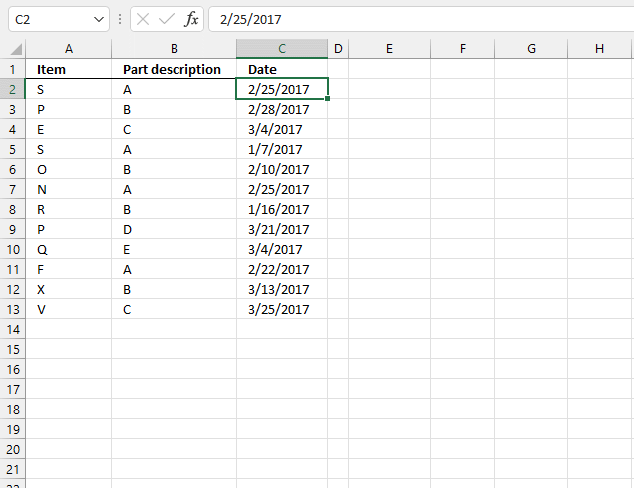### 14.1 VBA code

```'Name User Defined Function and define parameters
Function Lookup_concat(Search_Start As String, Search_End As String, _
Search_in_col As Range, Return_val_col As Range)

'Dimension variables and declare data types
Dim i As Long
Dim result As String

'Iterate through all cells in cearch range
For i = 1 To Search_in_col.Count

'Check if date is in date range
If Search_in_col.Cells(i, 1) <= Search_End And Search_in_col.Cells(i, 1) >= Search_Start Then

'Concatenate value from return range if date is in date range
result = result & Return_val_col.Cells(i, 1).Value & ", "
End If
Next

'Check if character length is larger than 0 (zero)
If Len(result) > 0 Then

'Remove last delimiting characters and return string to worksheet
Lookup_concat = Left(result, Len(result) - 2)

'Continue here date is not in date range
Else

'Return nothing to worksheet
Lookup_concat = ""
End If

End Function

```

### Get the Excel filelookup-within-a-date-range-and-return-concatenated-values.xlsm

## 15. Split search string using a delimiting character and return multiple matching values concatenated into one cell (UDF)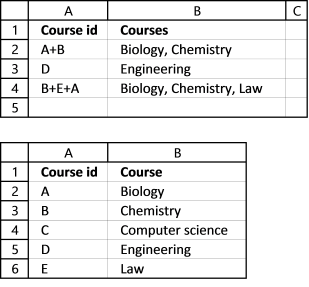This UDF lets you use multiple search strings and fetch corresponding values concatenated to one cell.

Example, search string in cell A2 (table 1) is A+B, the search delimiting character is +. The UDF looks for both A and B in column A in table 2. Cell A2 and A3 matches A and B so the values from column B (Biology and Chemistry) are retrieved and concatenated to cell B2.

Formula in cell B2:

=Lookup_concat(A3,"+",Sheet2!\$A\$2:\$A\$6,", ",Sheet2!\$B\$2:\$B\$6)

This is a User Defined Function so to use the above formula you need to first insert a few lines of code to your workbook.

### 15.1 VBA code

```'Name User Defined Function and define parameters
Function Lookup_concat(Search_string As String, Search_del As String, _
Search_in_col As Range, Concat_del As String, Return_val_col As Range)

'Dimension variables and declare data types
Dim i As Long, j As Long
Dim result As String
Dim srchArr() As String

'Split search string based on delimting character and save to array variable srchArr
srchArr = Split(Search_string, Search_del)

'Iterate through search range
For i = 1 To Search_in_col.Count

'Iterate through values in array variable srchArr
For j = LBound(srchArr) To UBound(srchArr)

'Check if value equals value in search range
If Search_in_col.Cells(i, 1) = srchArr(j) Then

'Concatenate value to variable result and a delimtiing character
result = result & Return_val_col.Cells(i, 1).Value & Concat_del
End If
Next j
Next

'Remove laste delimting characters and return string to worksheet
Lookup_concat = Left(result, Len(result) - Len(Concat_del))
End Function

```

Remember to save your workbook as an *.xlsm file or your code is lost the next time you open your workbook.

### Get the Excel fileplit-search-string-using-a-delimiting-character-and-return-multiple-matching-values-concatenated-into-one-cell.xlsm

## 17. Use multiple search values and return multiple matching values concatenated into one cell (UDF)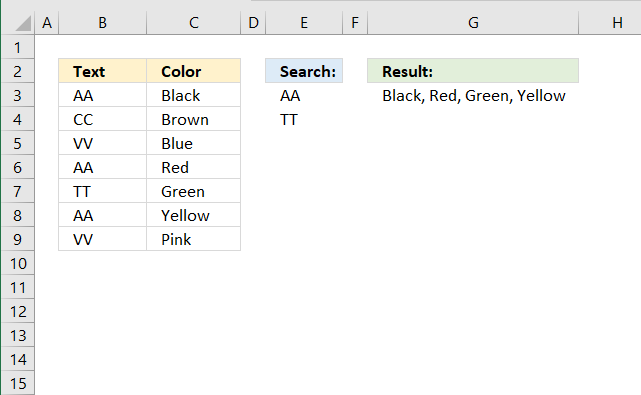Formula in cell G3:

=Lookup_concat(E3:E4,B3:B9,", ", C3:C9)

### 17.1 VBA Code

```'Name User Defined Function and define parameters
Function Lookup_concat(Search_string As Range, Search_in_col As Range, Concat_del As String, Return_val_col As Range)

'Dimension variables and declare data types
Dim i As Long, j As Long
Dim result As String

'Iterate through search range
For i = 1 To Search_in_col.Count

'Iterate through cells in parameter Search_string
For Each Value In Search_string

'Check if value is equal to cell in search range
If Search_in_col.Cells(i, 1) = Value Then

'Concatenate value to result and delimiting character
result = result & Return_val_col.Cells(i, 1).Value & Concat_del
End If
Next Value
Next i

'Remove last delimiting characters and return string result to worksheet
Lookup_concat = Left(result, Len(result) - Len(Concat_del))
End Function
```

#### 17.2 Get Excel *.xlsm file

Use multiple search values and return multiple matching values concatenated into one cell.xlsm